# Texas Go Math Grade 2 Lesson 5.4 Answer Key Use Ten to Subtract

Refer to our Texas Go Math Grade 2 Answer Key Pdf to score good marks in the exams. Test yourself by practicing the problems from Texas Go Math Grade 2 Lesson 5.4 Answer Key Use Ten to Subtract.

## Texas Go Math Grade 2 Lesson 5.4 Answer Key Use Ten to Subtract

Explore

Circle to show the amount you subtract for each problem.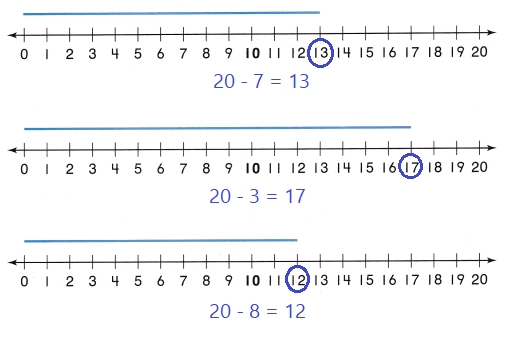Explanation:
To subtract the numbers on number line we move numbers to the left side of number line.

FOR THE TEACHER: Read the following problem. Scott has 13 crayons. He gives 3 crayons to Tyler. How many crayons does Scott have now? Have children circle the part of the blue line segment that shows what is subtracted from the total. Repeat for two more problems.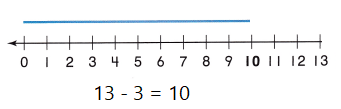Explanation:
Scott has 13 crayons.
He gives 3 crayons to Tyler.
Total number of crayons
13 – 3 = 10

Math Talk
Mathematical Processes

Describe a pattern in the three problems and answers.
In the above three problems total 20 counts are there
for the first problem
out of 20, blue line is drawn up to 13 count, the rest 7 blue line is not extended, then the answer is 20 – 7 = 13
for second problem
out of 20, blue line is drawn up to 17 count, the rest 3 blue line is not extended, then the answer is 20 – 3 = 17
Third problem
out of 20, blue line is drawn up to 12 count, the rest 8 blue line is not extended, then the answer is 20 – 8 = 12

Model and Draw

You can subtract in steps to use a tens fact.So, 14 – 6 = ____________
14 – 4 = 10
10 – 2 = 8

Share and Show

Show the tens fact you used. Write the difference.Question 1.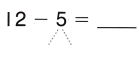10 – __________ = __________
Explanation:12 – 2 = 10
10 – 3 = 7

Question 2.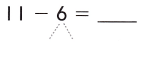10 – __________ = ___________
Explanation: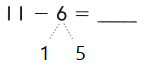11 – 1 = 10
10 – 5 = 5

Question 3.
15 – 7 = __________
10 – __________ = __________
Explanation: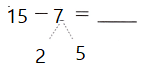15 – 5 = 10
10 – 2 = 8

Question 4.
13 – 7 = __________
10 – __________ = __________
Explanation:13 – 3 = 10
10 – 4 = 6

Problem Solving

Show the tens fact you used. Write the difference.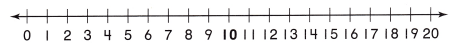Question 5.
13 – 5 = __________
10 – __________ = __________
Explanation: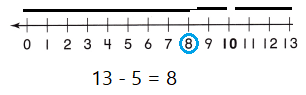13 – 3 = 10
10 – 2 = 8
Question 6.
15 – 6 = __________
10 – __________ = __________
Explanation: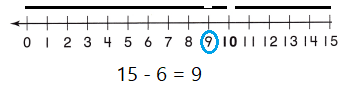15 – 5 =10
10 – 1  = 9

Question 7.
12 – 8 = __________
10 – __________ = __________
Explanation: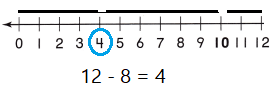12 – 2 = 10
10 – 6 = 4

Question 8.
14 – 8 = __________
10 – __________ = __________
Explanation:14 – 4 = 10
10 – 4 = 6

Solve. Write or draw to explain.

Question 9.
H.O.T. Beth has a box of 16 crayons. She gives 3 crayons  to Jake and 7 crayons to Wendy. How many crayons does Beth have now?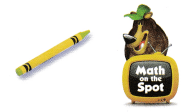_________ crayons
6 crayons
Explanation:
Beth has a box of 16 crayons.
She gives 3 crayons  to Jake and 7 crayons to Wendy3 + 7 = 10
16 – 6 = 10
10 – 4 = 6

Question 10.
H.O.T. Multi-Step Chris had 15 stickers. He gave Ann and Suzy each the same number of stickers. Now Chris has 7 stickers.
How many stickers did he give to each girl?__________ stickers
8 stickers
Explanation:
He gave Ann and Suzy each the same number of stickers.
Now Chris has 7 stickers.
Number of stickers he gave to each girl15 – 5 = 10
10 – 2 = 8

Question 11.
Analyze Tasho found two sea stars. The first sea star had 12 arms. The second sea star had 7 arms. How many more arms did the first sea star have?
(A) 6
(B) 5
(C) 9
Option (B)
Explanation:
Analyze Tasho found two sea stars.
The first sea star had 12 arms.
The second sea star had 7 arms.
Number of more arms did the first sea star have
12 – 7 = 5

Question 12.
Apply Mr. Cruz wants to buy 11 postcards. So far, he has found 8 postcards. How many more postcards does Mr. Cruz need to find?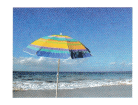(A) 10
(B) 11
(C) 3
Option (C)
Explanation:
Mr. Cruz wants to buy 11 postcards.
he has found 8 postcards.
Number of more postcards does Mr. Cruz need to find
11 – 8 = 3

Question 13.
Meg has 14 shells. That is 6 more shells than Lia has. How many shells does Lia have?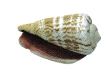(A) 6
(B) 20
(C) 8
Option (B)
Explanation:
Meg has 14 shells.
That is 6 more shells than Lia has.
Number of shells does Lia have in all
14 + 6 = 20

Question 14.
TEXAS Test Prep Andy scored 13 points in the first game and 7 points in the second game. How many more points did he score in the first game than in the second game?
(A) 6
(B) 4
(C) 20
Option (A)
Explanation:
Andy scored 13 points in the first game and
7 points in the second game.
Number of more points he score in the first game than in the second game
13 – 7 = 6

TAKE HOME ACTIVITY • Ask your child to name pairs of numbers that have a difference of 10. Then have him or her write the number sentences.
10 and 20 are two numbers that have a difference of 10
20 – 10 = 10

### Texas Go Math Grade 2 Lesson 5.4 Homework and Practice Answer Key

Show the tens fact you used. Write the difference.Question 1.
11 – 5 = __________
10 – __________ = __________
Explanation:11 – 1 = 10
10 – 4 = 6

Question 2.
14 – 7 = __________
10 – __________ = __________
Explanation: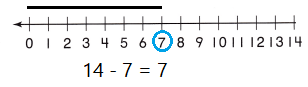14 – 4 =10
10 – 3 = 7

Question 3.
15 – 8 = __________
10 – __________ = __________
Explanation:15 – 5 = 10
10 – 3 = 7

Question 4.
18 – 9 = __________
10 – __________ = __________
Explanation: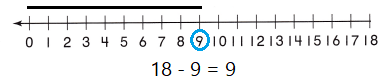18 – 8 = 10
10 – 1 = 9

Problem Solving

Solve. Write or draw to explain.

Question 5.
Joan has a box of 14 blue beads. She gives 5 beads to Judy and 5 beads to Sara. How many beads does Joan have now?
Explanation:
Joan has a box of 14 blue beads.
Number of beads does Joan have now
14 – 5 = 9
9 – 5 = 4

Question 6.
Multi-Step Jake had 17 toy cars. He gave Dan and Andy each the same number of toy cars. Now Jake has 5 toy cars. How many toy cars did he give to each friend?_________ toy cars
6 toy cars
Explanation:
He gave Dan and Andy each the same number of toy cars.
Now Jake has 5 toy cars.
Number of toy cars he give to each friend
17 – 12  = 5
6 toy cars did he give to each friend

Lesson Check

Question 7.
On Monday Lynn read 11 pages in her book. On Tuesday she read 9 pages in her book. How many more pages did she read on Monday than on Tuesday?
(A) 2
(B) 20
(C) 8
Option (B)
Explanation:
On Monday Lynn read 11 pages in her book.
On Tuesday she read 9 pages in her book.
Number of more pages she read on Monday than on Tuesday
11 + 9 = 20

Question 8.
Carolyn and Lee Ann are collecting pennies. Lee Ann found 16 pennies. That is 8 more pennies than Carolyn found. How many pennies did Carolyn find?
(A) 10
(B) 8
(C) 6
Option(B)
Explanation:
Lee Ann found 16 pennies.
8 more pennies than Carolyn found.
Number of pennies Carolyn find
16 – 8 = 8

Question 9.
Ben is buying sailboat pictures on his vacation. He wants to buy 12 different pictures. So for, he has bought 5. How many more sailboat pictures does he need to buy?
(A) 7
(B) 5
(C) 17
Option(A)
Explanation:
Ben wants to buy 12 different pictures.
He has bought 5.
Number of more sailboat pictures he need to buy
12 – 5 = 7

Question 10.
Sam has two bags of marbles. The first bag has 13 marbles. The second bag has 9 marbles. How many more marbles does the first bag of marbles have?
(A) 3
(B) 5
(C) 4